# CH 105 - Chemistry and Disease

### Stoichiometry

04/04/2005

Moles, Molar Mass, and Avogadro's Number

To understand how substances react chemically, we need to be able to determine how many molecules of one substance reacts with another substance. In the lab we do not measure molecules directly but rather the mass of the reacting chemicals and products. Therefore we have to be able to convert between mass in grams and molecules.

I started off the a simple demonstration of 4 different fruits (a raisin, grape, apple, and grapefruit) in sealed boxes.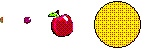If each box contained the same number of pieces of fruit, then the box with the fruit with the heaviest piece of fruit would obviously weigh more. Converse, if the mass of the boxes were the same, then the box with the lightest piece of fruit would have the most pieces of fruit. If you knew the weight of each piece of fruit, you could determine the number of pieces of fruit in the box.

Now consider atoms like He, C, or Ti, and a molecule like sulfuric acid, H2SO4. They are arranged in term of increasing atomic weight (or molecular weight for sulfuric acid). Hence if different boxes contained equal number of these different atoms or molecules, then the box with sulfuric acid would weight most, and the box with He would weigh least. Conversely, if the boxes weighed the same amount, the box containing the lightest atom, He, would contain more particles than the others.

Lets go back to the periodic table

 Group Period 1A 2A 3B 4B 5B 6B 7B 8B 9B 10B 11B 12B 3A 4A 5A 6A 7A 8A 1 1 H 1.01 2 He 4.00 2 3 Li 6.94 4 Be 9.01 5 B 10.81 6 12.01 7 14.01 8 16.00 9 19.00 10 20.18 3 11 22.99 12 24.31 13 Al 26.98 14 Si 28.09 15 P 30.97 16 32.07 17 35.45 18 Ar 39.95 4 19 39.10 20 40.08 Sc Ti V Cr Mn Fe Co Ni Cu Zn Ga Ge As Se Br Kr 5 Rb Sr Y Zr Nb Mo Tc Ru Rh Pd Ag Cd In Sn Sb Te I Xe 6 Cs Ba La Hf Ta W Re Os Ir Pt Au Hg Ti Pb Bi Po At Rn 7 F Ra Ac Rf Db Sg Bh Hs Mt Uun Uuu Uub
 Atomic # Symbol Avg. Atomic Mass

Lets look at carbon.  Its atomic number is 6 (meaning it has 6 protons and 6 electrons in the neutral atom).  It has an average atomic mass of 12.01.  What are the units? Grams? Pounds? Tons?  Obviously not.  Notice that H has an atomic mass of 1.01.  Whatever the units are, that means that an atom of C has a 12 times the mass of an atom of H.  The mass derives mostly from neutrons and protons, and we neglect the contribution from electrons.  H has 1 proton and 0 neutrons, while C has 6 protons and 6 neutrons (in its most common form, although there are some rarer "isotopes"  that have more neutrons ).  The mass unit we use is the atomic mass unit (AMU).  The H atoms (with one proton) has a mass of 1 AMU.   Carbon with 6 protons and 6 neutrons has mass of 12 AMU.

Now if we had two boxes with identical masses, one containing H atoms and one C atoms, we could say that there are more atoms in the H box than in the C box.  Likewise if the box containing C atoms had 12 times the mass of the box containing the H atoms, we could say that they had an equal number of atoms in each box.

In our lab, we can't determine that mass of a few atoms of a substance.  However, we can easily measure 1 g of H atoms and 12 g of C atoms  (assuming that the atoms didn't chemically react with each other and were stable, which we know they aren't). In these two boxes, we would have the same number of atoms. How many? A very large number called Avogadro's  Number which is 6.022 x 1023 atoms.  We also define another term since that number is much to big to use routinely.  We define an amount of an element  which contains 6.022 x 1023 atoms of that element, or in the case of C and H, 12 and 1g, respectively, as one mole of that element.

The atomic weight of an atom of a pure element like Cu, Ti, etc, or the molecular weight of a molecule (calculated by adding up the atomic weights of all of its atoms), both expressed in grams, contains 6.022 x 1023 atoms or molecules of that substance. or one mole.   The molar mass is the atomic (for atoms) or molecular weight (for molecules) expressed in grams. The "amount" of a substance that contains 6.022 x 1023 particles of that substance is 1 mole of that substance. You must be able to convert, using the factor-label method to convert between moles, molar mass, and number of molecules.

It just so happens that 12.0 g of 12C has 6.022 X 1023 atoms. Too bad it is such a bizarre number. It would have been better if it were 1 billion, but there is nothing any of us can do about it. We define 1 mole of 12C as the mass of 12C that contains 6.022 X 1023 atoms., which , as described above, has a mass of 12.0 g. An atom of H has a mass 1/12 of carbon. (Look at the atomic weights) Therefore 6.022 X 1023 atoms of H would weight 1/12 of 12.0 g, or 1.0 g, which again happens to be the atomic weight of H expressed in grams. Likewise, H2O (molecular weight of 18.0) has a molar mass of 18.0 g. 18.0 g of water is 1 mole of water and contains 6.022 X 1023 molecules of water. Hemoglobin (MW = 64,000) has a molar mass of 64,000 g. 64,000 g of hemoglobin contains 6.022 X 1023 molecules of hemoglobin. By the way 1 mole of elephants would be 6.022 X 1023 elephants.

Interpreting Chemical Equations

In class we wrote and balanced a chemical equation for the reaction of gold sulfide (Au with a 3+ charge) with hydrogen gas (H2).  The balanced equation below illustrates the important features of the equations

Au2S3 + 3 H2 ------> 2 Au + 3 H2S

This chemical equation can be translated into English as follows:

1 molecule of gold sulfide reacts with 3 molecules of hydrogen to produce 2 atoms of gold plus 3 molecules of hydrogen sulfide.

Both sides of a chemical equation, like a mathematical equation, can be multiplied by the same number. The table below shows various amounts of reactants and products that are obtained by multiplying the chemical equation by some number.

 multiply by Au2S3 3 H2 2 Au 3 H2S - 1 molecule 3 molecules 2 atoms 3 molecules 2 2 molecules 6 molecules 4 atoms 6 molecules 12 12 molecules 36 molecules 24 atoms 36 molecules OR 1 DOZEN 3 DOZEN 2 DOZEN 3 DOZEN 6.02 X 1023 1(6.02 X 1023) molecules 3 (6.02 x 1023) molecules 2(6.02 x 1023) atoms 3(6.02 x 1023) molecules OR 1 mole molecules. 3 moles molecule 2 moles atoms 3 moles molecule which equals 490 g 6.0 g 394 g 102 g

Please note the following:

• You must change all the coefficients of the equation in the same proportions. You can not just change one of them.
• The total number of mole on the left hand side does not necessarily equal the total number of moles on the right hand side.
• The total mass on the left hand side is equal to the total mass on the right. This must be true if the Law of Conservation of Mass is true.

Stoichiometry

The coefficients in front of balanced chemical equations tell us the number of moles of reactants that react to form a specific number of moles of products. The reaction stoichiometry is the quantitative relations between the amount of reactants consumed and products formed in chemical reactions as depicted in the balanced chemical equations. Stoichimetric relationship can be used to determine that in the chemical reaction below :

Au2S3 + 3 H2 --> 2 Au + 3 H2S

490 g of Au2S3 reacts with 6 g H2 to give 394 g of Au and 192 g of H2S. (See table above). Simple proportions could be used to determine amounts of gold and hydrogen sulfide produced if the reactants were present at half the amounts listed above.

What would happen, however, if we had 490 g of gold sulfide and 100 g of hydrogen?   Or a million g of hydrogen?  Clearly not all the hydrogen could react. Hydrogen is in excess and some would be left after the reaction. Gold sulfide is the limiting reagent and the amount of products produced would be determined only by the amount of the gold sulfide, and not the hydrogen.

A simple example of the concept of excess/limiting reagents is illustrated in the model below. You have two different 2D structures, a triangle and a square with a triangular indentation the same size of the triangle. You have 1 square and 4 triangles.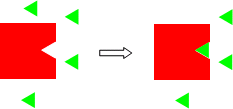The triangular indentation is lined with Velcro, and the triangle with the opposite type of Velcro. Now mix them together. Since only one triangle can fit into the indentation in the square, after mixing one square-triangle "complex" results and 3 triangles are left over. For an obvious chemical example, burn a 10 g piece of coal that is pure carbon in the air. Obviously oxygen in the air is in huge excess and the coal is limiting.

CONCENTRATION

The concentration of a solution is the mass (in grams) of a substance that is dissolved in a given volume (in cubic centimeters or cm3) of solution. The substance that is dissolved can be a solid (like salt), a liquid (like vinegar), or a gas (like oxygen or carbon dioxide). Concentration can be found by dividing the mass of the subtance dissolved by the total volume of solution. This can written in a formula:

concentration = mass of a dissolved substance/volume of solution.

The units of concentration are then grams/cubic centimeter or grams/cm3. People use concentration when they are talking about solutions. In a way it is analogous to another concept with which students have trouble - density.

DENSITY: What is density. It has to do with both the mass of an object and the volume of an object, just like concentration has to do with the mass of a dissolved sustance and the volume of the solution.

DENSITY IS LIKE CONCENTRATION:

• density is mass substance per volume of the substance
• concentration is mass of a dissolve substance per volume of solution
• both have the same units - g/cm3

Peole use density when they want to determine the total mass of an object in a certain volume of that object. The object can be anything: a solid, a liquid, a gas, a mixture, a solution. The density of an object equals its mass divided by its volume. It has units of grams/cm3 .

density = mass of an object /volume of the object. Consider for a moment a cube with each side being 1 cm.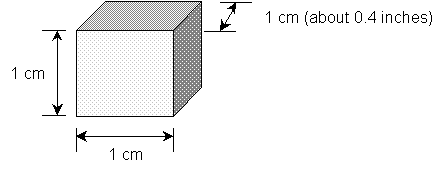Now it's easy to measure the mass of this object. Place the object on a scale or balance. The volume of the cube can be calculated by the simple formula.
Volume = V = length X width X height = 1 cm X 1 cm X 1 cm
1 cubic centimer = 1 cm3.

It's also easy to calculate the volume of an object if it is a simplegeometric shape. If it has a more complicated shape, you can measure its volume indirectly by putting it into a container with water. Look at the picture below.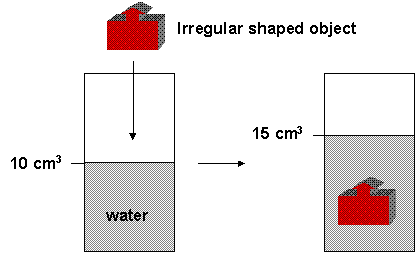What is the volume of the object? The total volume in the container with the object is equal to the volume of the water plus the volume of the solid. Hopefully you can tell that the volume of the object is 5 cm3. For this to work, the object can not dissolve. You can calculate the volume by putting an insoluble solid into water and determining the volume change of the water.

Now you have the mass of the object and its volume so you can calculate the density. Again, the units of density is grams/cubic centimeter.

EXAMPLE OF DENSITIES: What is the density of a cubic centimeter of a substance? Check out the chart below which shows the density of various subtances.

 SUBSTANCE PHYSICAL STATE DENSITY (g/cm3) hydrogen gas 0.000090 oxygen gas 0.00143 stryfoam solid about 0.08 alcohol liquid 0.785 water liquid 0.998 salt solid 2.16 aluminum solid 2.70 copper solid 8.96 lead solid 11.34 gold solid 19.32.

Now for some really strange numbers. People have estimated the density of outer space, with its stars, planet, comets, and mostly "empty" space. The average density of space is about 3 x 10-24 or
0.000000000000000000000003 g/cm3 which should tell you that it really is mostly empty. Now at the opposite end, there are some incredibly dense stars called neutron stars. The stars have an unbelievably high density of about 1 x 1015 or 1,000,000,000,000,000 g/cm3.. To get the same density you would have to compress a cube of water with sides of 0.6 miles into a cube 1 cm on each side!

You can increase the volume of a substance by compressing it into a smaller volume. If you had a room full of feathers that might have a mass of 1000 grams, and you squash them into a smaller shape, what changes? The total mass doesn't but the volume get smaller. Since density is equal to mass divided by the volume, the density gets bigger. The picture below shows how decreasing the volume of an object increases its density.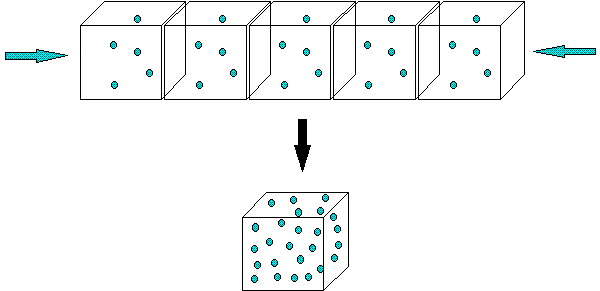The same diagram could represent a solution. You can increase the concentration of a solution by evaporating the water (but not the dissolved substance). The mass of the dissolved substances remains the same, but the volume it dissolves in decreases. Therefore, its concentration goes up. Hopefully you can see the similarities and differences between concentration of a solution and the density of a substance!

ANIMATION: Solution of Increasing Concentration

Expressing Solution Concentrations:

There are different ways to express concentration of a solute dissolved in a solvent. In each way, concentration is expressed as the amount of solute (g, ml,)dissolved in a given amount (g, ml) of SOLUTION. We will consider two different ways to determine concentration - % concentration and molarity

% concentration (w/w, v/v, or w/v): which can be any of the following

• weight solute/weight solution (w/w) - (g solute/100 g solution)100
• volume liquid solute/volume solution (v/v) - (mL solute/100 mL solution)100
• weight solute/volume solution (w/v) - (g solute/100 mL solution)100 - most common of the three types

Molarity (M)

• moles solute/L solution which is equivalent to
• mmole solute/mL solution.

The later statement can be seen from the following conversion:

1 M = 1 mol/L = 1 mol/L X 1000 mmol/mol X 1L/1000 mL = 1 mmol/mL

Hence a 0.1 M NaCl solution has 0.1 mole NaCl/L solution or 0.1 mmol NaCl/ml solution. In an analogous fashion 10 g/L = 10 mg/ml

% concentration is used if your main interest is knowing how much solute is in a given amount solution. Molarity units are most often used when you wish to determine changes in the the amounts and concentrations of reactants and products in a chemical reaction that occurs in solution.

To make a solution of a given % concentration or molarity, a given amount of solute is added quantitatively (using a balance to measure mass or a volumetric pipet to measure volume) to a solvent, the solute dissolved, and then transfered quantitatively to a volumetric flask. Solvent is added to a given final volume of total solution. Solutions can then be diluted to make less concentrated solutions. Dilutions of 1 --> 10 (10 fold dilution), 1 --> 100 (one hundred fold dilution) or 1 --> 1000 (thousand fold dilution) are often conveniently made. To make a 1000 fold dilution, you can take 1 mL of the stock solution, add it quantitativley to a 1 L volulmetric and add solvent to a volume of 1 L.

Since most reactions that we will deal with in this class occur in solution, we need a easy way to determine the amount or reactants used and products consumed. Consider the following two reactions:

1. NaCl(aq) + AgNO3(aq) ---> NaNO3(aq) + AgCl(s)
2. HCl(aq) + NaOH(aq) --> NaCl(aq) + H2O(l)

In both of these reactions 1 mole of each reactant reacts to form 1 mole of each product. When dealing with aqueuous solutions, we usually know the molarity of the solution and the volume of each reactant solution. The above equations dont say the 1 ml of each reactant or 1 g of each reactant forms 1 ml or 1 g of each product. Rather it states that number of moles of reactants and products. Unit analysis shows that for any given reactant or product:

Molarity x Volume = M x Vol = Mol/L x L = moles. Or using easier units:

Molarity x Volume = M x Vol = mMol/mL x mL = mmoles

Given two of the following qualities (M, V, moles) you should be able to detemine the other quantitiy, since M = mol/V. Likewise you should be able to convert from M = mol/L = mmol/ml to g/L = mg/ml.

In equation 2 above, 1 mole of HCl reacts with 1 mole of NaOH to form 1 mole of NaCl and 1 mole of H2O. If you are given a volume and molarity of a given solutions, you must convert these units to moles, since the chemical equations describes the number of moles. Then you can determine the number of moles of product, which can be converted to a volume or molarity. Given 2 of the following quantities, you can get the other: Molarity, moles, volume.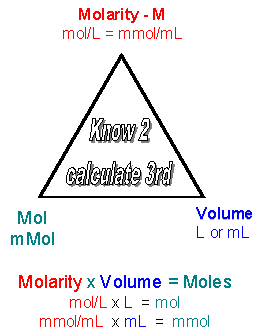Practice solution concentrations, dilution, and stoichiometry calculations in the quiz below.

WCB QUIZ: Solution Concentration and Stoichimetry

WCB QUIZ: Solution Concentration and Stoichimetry - ANSWERS